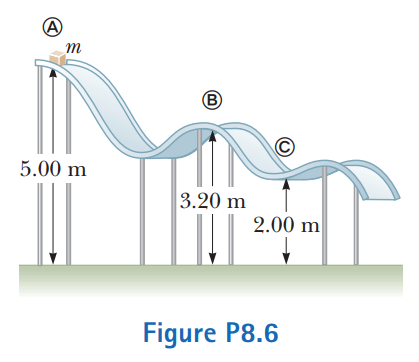# Problem: A block of mass m = 5.00 kg is released from point A and slides on the frictionless track shown in Figure P8.6. Determine(a) the block’s speed at points B and C and(b) the net work done by the gravitational force on the block as it moves from point A to point C.

###### FREE Expert Solution

This is an energy conservation problem. We'll set up the energy conservation equation. The rest is plug-chugg!

Energy conservation equation:

95% (16 ratings)###### Problem Details

A block of mass m = 5.00 kg is released from point A and slides on the frictionless track shown in Figure P8.6. Determine(a) the block’s speed at points B and C and

(b) the net work done by the gravitational force on the block as it moves from point A to point C.

Frequently Asked Questions

What scientific concept do you need to know in order to solve this problem?

Our tutors have indicated that to solve this problem you will need to apply the Motion Along Curved Paths concept. You can view video lessons to learn Motion Along Curved Paths. Or if you need more Motion Along Curved Paths practice, you can also practice Motion Along Curved Paths practice problems.

What professor is this problem relevant for?

Based on our data, we think this problem is relevant for Professor Rybolt's class at KSU.

What textbook is this problem found in?

Our data indicates that this problem or a close variation was asked in Physics for Scientists and Engineers - Serway Calc 9th Edition. You can also practice Physics for Scientists and Engineers - Serway Calc 9th Edition practice problems.